# Quantum Simulation of Gauge Theoriesin collaboration with Andrei Alexandru, Paulo Bedaque, Siddhartha Harmalkar,
Hersh Kumar, Henry Lamm, Neill Warrington, Yukari Yamauchi"The best model of a cat is another cat"
Norbert Wiener

# Neutron stars and little bangs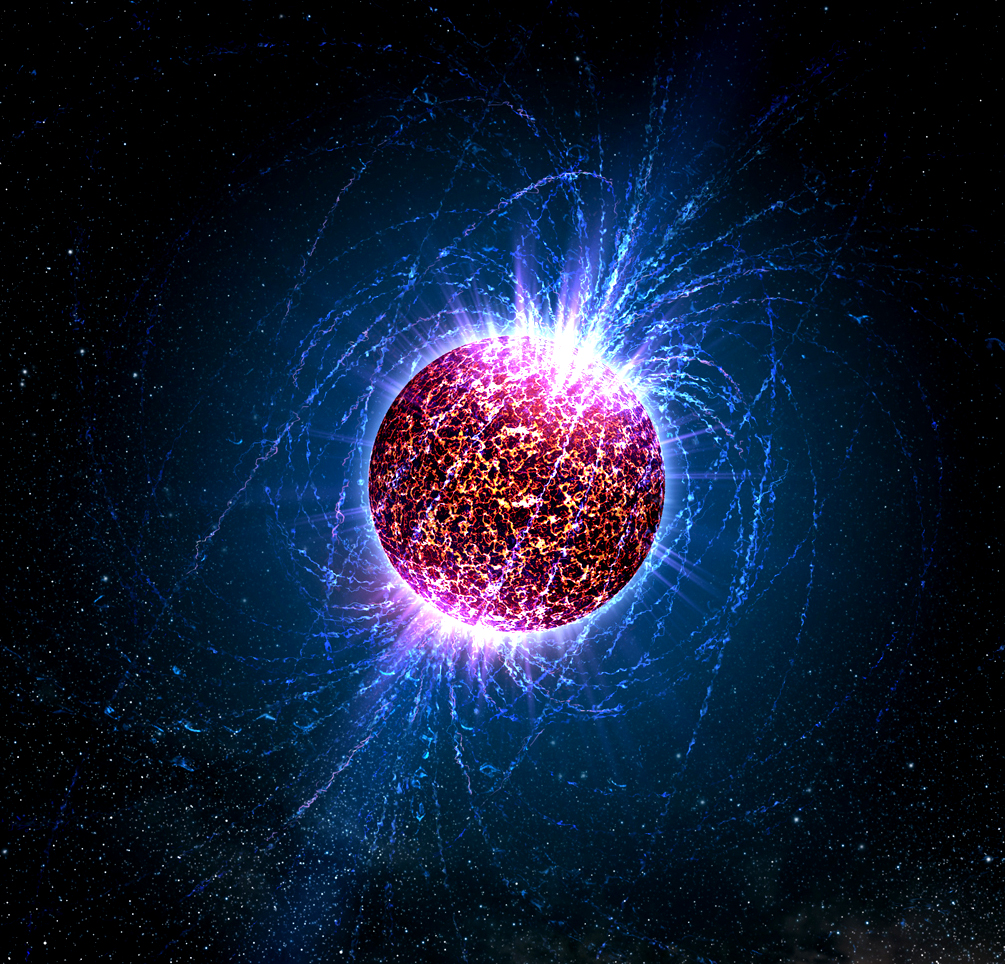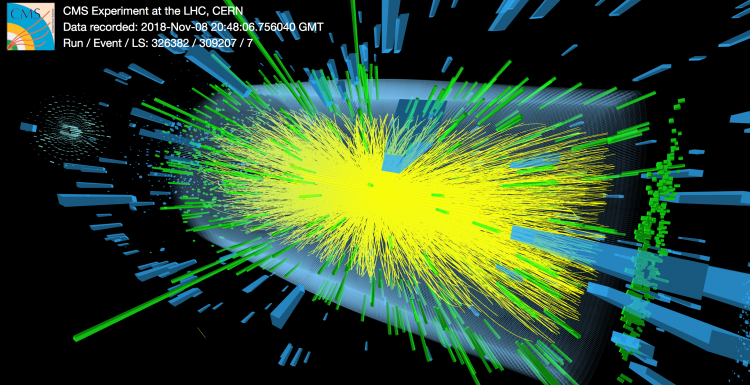Thomas McCauley/CERN
$$\mathscr L_{\mathrm{\color{red}Q\color{green}C\color{blue}D}} = - \frac 1 4 F_{\mu\nu} F^{\mu\nu} + \bar \psi \left(i \gamma^\mu D_\mu - m\right)\psi$$

# The lattice, briefly

QCD is a non-perturbative theory. (There's no small parameter about which to Taylor expand.)

At every link lives a unitary matrix. $$U_{yx} \in SU(3)$$

The action is a sum over all `plaquettes'. $$S = \sum_P \mathrm{Re\,Tr\,} P$$This is a theory of with a finite number of degrees of freedom. Expectation values are given by: $$\left<\mathcal O\right> = \frac{\int \mathcal D U\;e^{-S[U]} \mathcal O[U]}{\int \mathcal D U\;e^{-S[U]}}$$

# A sign problem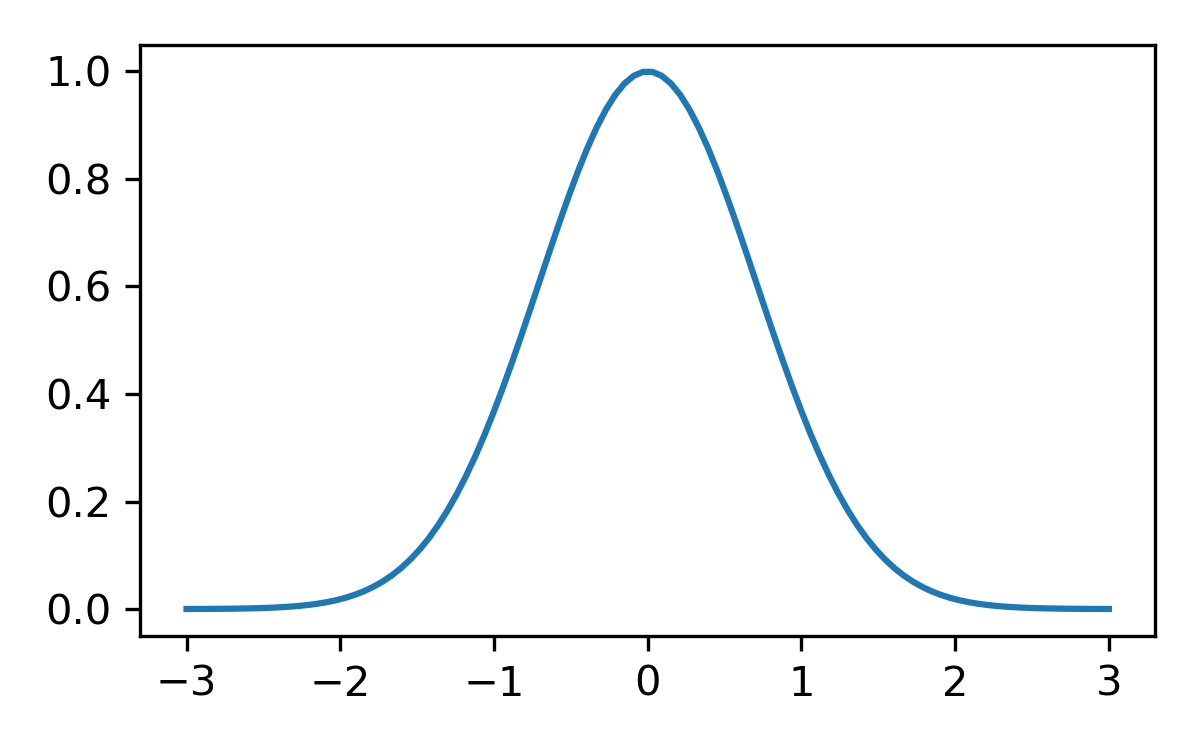$$\int f \approx 1 \pm 0.5$$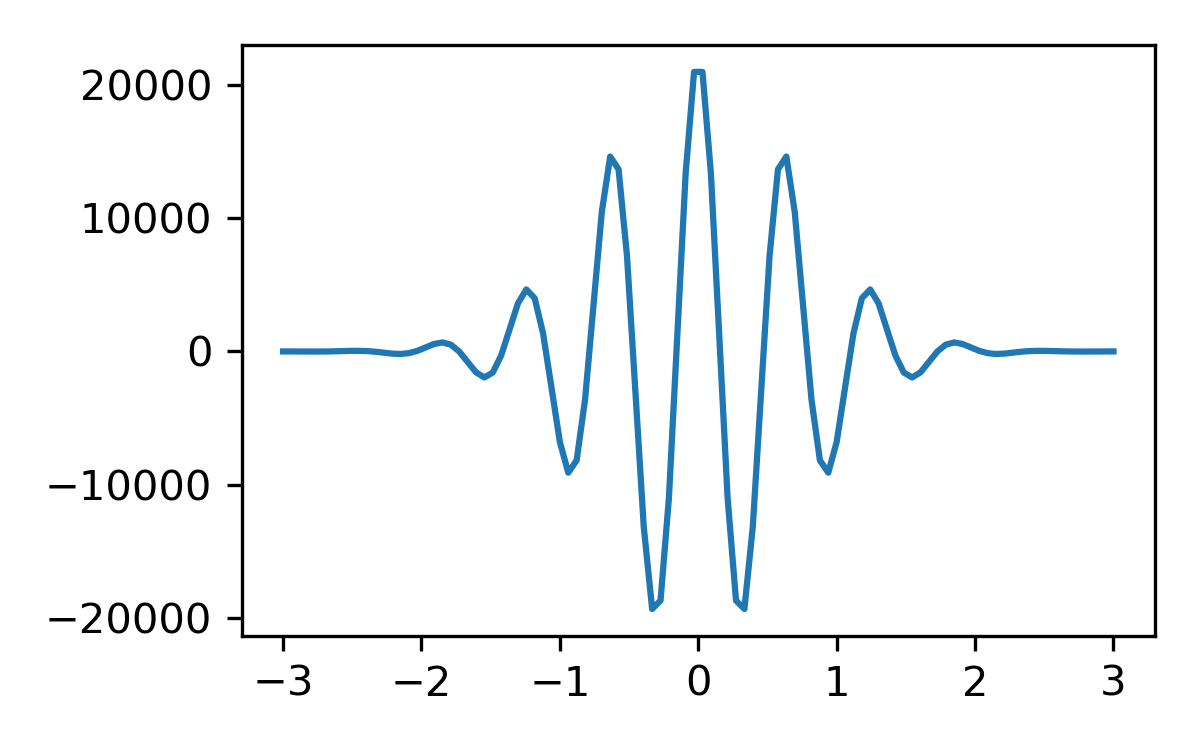$$\int f \approx 0 \pm 10^4$$

$$\langle x_1|e^{-i H t}|x_0\rangle = \int_{x(0)=x_0}^{x(1)=x_1} \mathcal D x(t) \;e^{i S[x]}$$
$$x(t)$$
$$\mathrm{Re\,}e^{iS[x]}$$

# What calculations have a sign problem?

Calculations that have sign problems:

• Real-time response (as opposed to thermal equilibrium)
• Hubbard model away from half-filling
• Finite density of relativistic fermions

Closely related, but mild: determining the mass of a proton

Calculations without sign problems:

• Zero-fermion-density equation of state
• Some masses (pion)

# Overview

The best model of a quantum system is another quantum system.
Norbert Wiener, almost
A quantum computer is a quantum system evolved in real-time.

Set up an analogy between the quantum computer and the system to be simulated, and treat the computer like a (perfectly controlled) laboratory.

• Some quantum algorithms
• Hamiltonian lattice gauge theory
• Quantum simulations of field theory
• Hybrid algorithm for near-term quantum computers

# From Bit to Qubit

$$|0\rangle,|1\rangle,\ldots,\frac{1}{\sqrt{2}}\left(|0\rangle+|1\rangle\right)$$This is a spin from QM.# A Quantum ComputerWhat's the Hilbert space? $$\mathcal H = \mathcal H_1 \otimes \cdots \otimes \mathcal H_1 \;\text{ where } \mathcal H_1 = \mathrm{span}\{|0\rangle,|1\rangle\}$$

In other words, superpositions of all possible bitstrings. $$\mathcal H = \mathrm{span}\{|00\cdots\rangle,|10\cdots\rangle,|01\cdots\rangle,\cdots\}$$

$$\left({\color{blue}|0\rangle + i|1\rangle}\right)\left({\color{green}|0\rangle+|1\rangle}\right) = |{\color{blue}0\color{green}0}\rangle + |{\color{blue}0\color{green}1}\rangle + i|{\color{blue}1\color{green}0}\rangle + i|{\color{blue}1\color{green}1}\rangle$$ Not entangled
$$|{\color{blue}0}{\color{green}0}\rangle + |{\color{blue}1}{\color{green}1}\rangle$$ Entangled

# Gates

All operations must be unitary (conservation of probability). On one qubit:

$$T = \left( \begin{matrix} 1 & 0\\ 0 & e^{i \pi / 4}\\ \end{matrix} \right)$$
$$H = \frac 1 {\sqrt{2}}\left( \begin{matrix} 1 & 1\\ 1 & -1\\ \end{matrix} \right)$$

On two qubits, the controlled-not operation:

$$CX = \left( \begin{matrix} 1 & 0 & 0 & 0\\ 0 & 1 & 0 & 0\\ 0 & 0 & 0 & 1\\ 0 & 0 & 1 & 0\\ \end{matrix} \right) \left( \begin{matrix} |00\rangle\\ |01\rangle\\ |10\rangle\\ |11\rangle\\ \end{matrix} \right)$$

These fundamental gates are sufficient to construct any unitary we want.

# Measurement

In principle, we measure any Hermitian operator. In practice, we measure $$\sigma_z$$ acting on each qubit. $$\Psi = \alpha|0\rangle + \beta|1\rangle$$

Each measurement yields $$1$$ or $$0$$. Probability of $$1$$ is $$\langle\Psi|1\rangle\langle 1|\Psi\rangle = |\beta|^2$$.

Thus, we require many measurements for a precise result. This is "shot noise".

Hermitian operators are exactly those which may appear as terms in the Hamiltonian.

# State of the art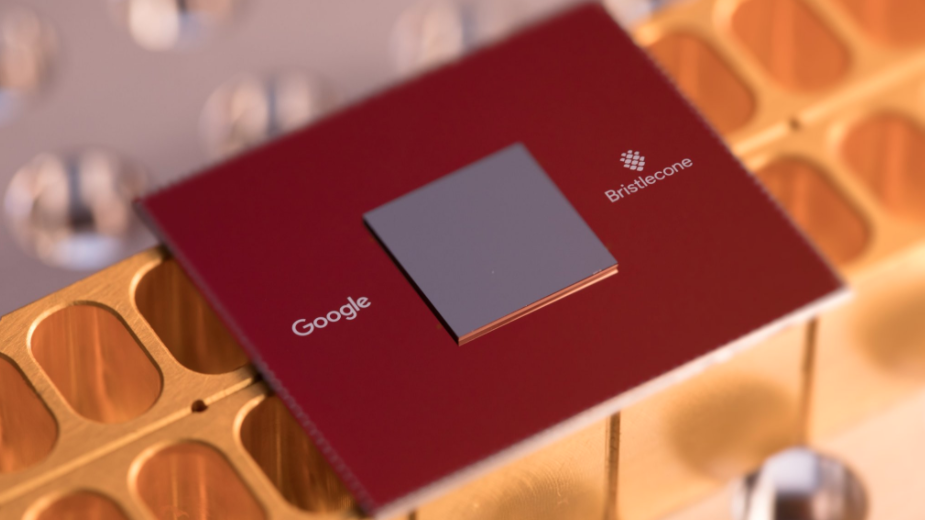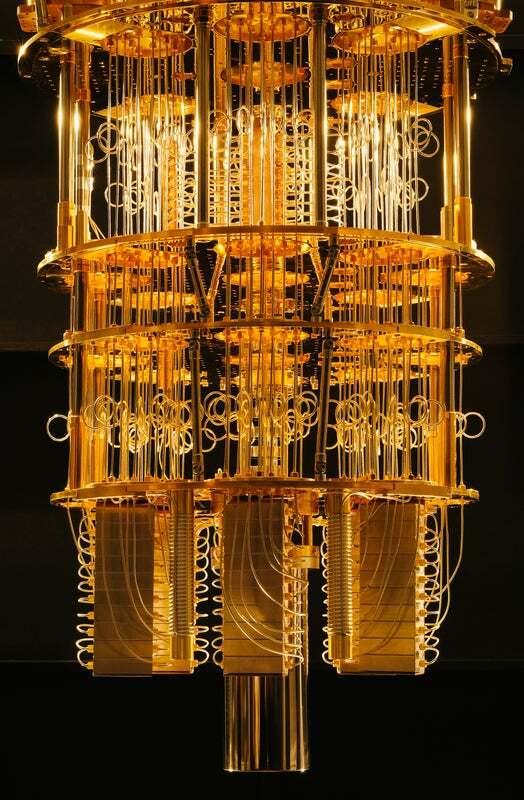Current best: $$\sim 50$$ qubits.

Each qubit can undergo $$\lesssim 10$$ operations before decohering.

Are large processors worth it?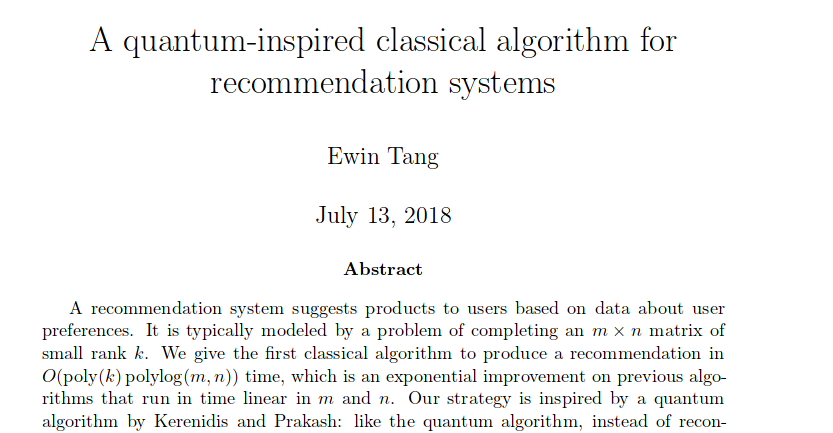# What is a classical computer?

A quantum computer constantly being measured
$$|01\rangle$$ is okay; $$\left[|10\rangle + |01\rangle\right]$$ gets destroyed

This restricts the set of possible operations, as well. We only have permutations:

$\left( \begin{matrix} 1& 0 & 0 & 0\\ 0& 1 & 0 & 0\\ 0& 0 & 0 & 1\\ 0& 0 & 1 & 0\\ \end{matrix} \right)$ and not $\left( \begin{matrix} -i & 0\\ 0 & i\\ \end{matrix} \right)$

# Classical algorithms are quantum algorithms

Any classical circuit can be re-labelled as a quantum circuit.

Example: two-bit adder \begin{align} |00\rangle |00\rangle &\rightarrow |00\rangle |00\rangle \\ |01\rangle |00\rangle &\rightarrow |01\rangle |01\rangle \\ |10\rangle |00\rangle &\rightarrow |10\rangle |01\rangle \\ |11\rangle |00\rangle &\rightarrow |11\rangle |10\rangle \\ \end{align}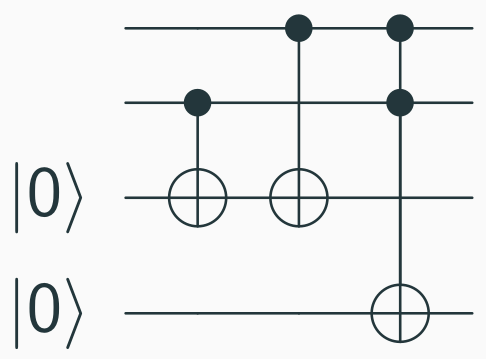In general, given a classical circuit for $$f(x)$$, we can obtain $$|x\rangle|0\rangle \rightarrow |x\rangle |f(x)\rangle$$

# Suzuki-Trotter decomposition

$$H = {\color{blue}A} + {\color{green}B}$$

Assume we can evolve under $$A$$ and $$B$$. How to get evolution under $$H$$? $$e^{-i H \epsilon} \approx {\color{blue}e^{-i A \epsilon}} {\color{green}e^{-i B \epsilon}}$$

So, time-evolve by rapidly alternating between two Hamiltonians $$e^{-i H t} \approx \left({\color{blue}e^{-i A \epsilon}} {\color{green}e^{-i B \epsilon}}\right)^{t / \epsilon}$$

# Field theories, the finite wayEach lattice site has a degree of freedom with Hilbert space $$\mathcal H_1$$. The whole system has Hilbert space $$\mathcal H = \mathcal H_1 \otimes \mathcal H_1 \otimes \cdots$$

Some Hamiltonian $$H$$ couples the different lattice sites. For a spin system, we might have $$H = \sum_{\langle i j\rangle} \sigma_z(i) \sigma_z(j) + \sum_i \sigma_x(i)$$

When correlations are large, the lattice structure is irrelevant, hence "field theory".

# Quantum mechanics on a group

The Hamiltonian of a free particle moving on $$G = SU(3)$$: $$H = -\nabla_\ell^2$$

Hilbert space is $$\mathbb C G$$, the space of complex functions on $$G$$.

$$\left|\left( \begin{matrix} 1 & 0 & 0\\ 0 & 1 & 0\\ 0 & 0 & 1\\ \end{matrix} \right)\right>, \left|\left( \begin{matrix} 0 & 1 & 0\\ 0 & 0 & i\\ -i & 0 & 0\\ \end{matrix} \right)\right>, \left|\left( \begin{matrix} 0 & 0 & 1\\ \frac{1}{\sqrt{2}} & \frac{1}{\sqrt{2}} & 0\\ \frac{1}{\sqrt{2}} & \frac{-1}{\sqrt{2}} & 0\\ \end{matrix} \right)\right>$$

We can work with momentum eigenstates instead.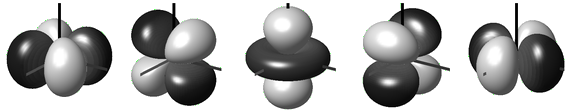wikimedia

# Gauge theories$$H = -\beta_K\sum_\ell \nabla_\ell^2 + \beta_P\sum_P \mathrm{Re\,Tr\,}P$$

# Gauge symmetry$$U_{ij} \mapsto V_j U_{ij} V_i^\dagger$$
\begin{align} &\mathrm{Tr\,} U_{14}^\dagger U_{45}^\dagger U_{25} U_{12}\\ \mapsto& \mathrm{Tr\,}U_{14}^\dagger U_{45}^\dagger U_{25} V^\dagger_2 V_2 U_{12} \end{align}

The Hamiltonian is gauge-invariant. $$H = -\beta_K\sum_\ell \nabla_\ell^2 + \beta_P\sum_P \mathrm{Re\,Tr\,}P$$

# Hilbert space$$\mathcal H = \mathbb C G \otimes \mathbb C G \otimes \cdots$$
Only gauge-invariant states are physical.
$$\color{red}|U_{12}\rangle$$ $$\color{green} \int \mathrm{d} V_1 \mathrm{d} V_2 |V^\dagger_{2} U_{12} V_1\rangle$$

Here's a projection operator: $$P |U_{12}\cdots\rangle = \int(\mathrm{d}V_1 \mathrm{d}V_2\cdots)|(V^\dagger_2 U_{12} V_1)\cdots\rangle$$

# Example: $$\mathbb Z_2$$ gauge theory$$H = \sigma_x(a) + \sigma_x(b) + \sigma_z(a) \sigma_z(b)$$

Four states, naively: $$|00\rangle$$, $$|01\rangle$$, $$|10\rangle$$, $$|11\rangle$$. But these are not gauge-invariant!

Gauge transformation takes $$00 \leftrightarrow 11$$ and $$01 \leftrightarrow 10$$. $$\color{green} |00\rangle + |11\rangle \;\text{ and }\; |01\rangle + |10\rangle$$

# Simulating a field theory: general principlesWe need a mapping between the Hilbert spaces. Locality is nice. $$\mathcal H_1 \leftrightarrow \mathcal H_1^{\mathrm{QC}}$$

This induces a "nice" map $$\mathcal H \leftrightarrow \mathcal H^{\mathrm{QC}}$$

Implement $$e^{-i H t}$$ via Suzuki-Trotter

# Time evolution

$$H = {\color{red}\overbrace{\sum_L \nabla^2_L}^{H_K}} + {\color{blue}\overbrace{\sum_P \mathrm{Re\,Tr\,} P}^{H_V}}$$
Kinetic

Diagonal in Fourier space

Mutually commuting terms

Potential

Diagonal (in our basis)

Mutually commuting terms

$e^{-i H t} \approx \left[{\color{red}\left( e^{-i \nabla_1^2 \epsilon} e^{-i \nabla_2^2 \epsilon} \cdots \right)} {\color{blue}\left( e^{-i \epsilon \mathrm{Re\,Tr\,} P_1} e^{-i \epsilon \mathrm{Re\,Tr\,} P_2} \cdots \right)} \right]^{t/\epsilon}$

# Example: $$\mathbb Z_2$$ gauge theory (again)$$H = \sigma_x(a) + \sigma_x(b) + \sigma_z(a) \sigma_z(b)$$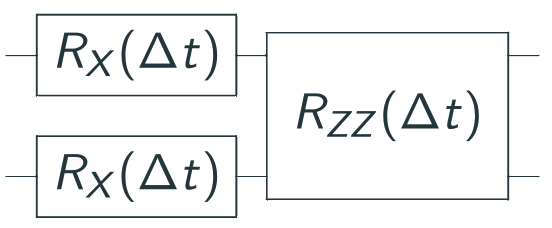# Valentiner gauge theory

We'd like to simulate $$SU(3)$$, but $$\mathbb C SU(3)$$ is infinite-dimensional.$\color{red}2^Q < \infty$
We can approximate $$SU(3)$$ by a finite subgroup.
$S(1080) < SU(3)$

# Gauge invariance

The physical Hilbert space is not $$\mathbb C S(1080) \otimes \mathbb C S(1080) \otimes\cdots$$.

But, the Hilbert space on the quantum computer is isomorphic to that space!

Time evolution is gauge-invariant.
$$[P, H] = 0$$

If we start in a gauge-invariant state, we stay gauge-invariant.

# One type of noise

Coherent noise that violates gauge invariance$$\color{green} |0_+\rangle = |00\rangle + |11\rangle$$ $$\color{red} |0_-\rangle = |00\rangle - |11\rangle$$
$$U_{\mathrm{drift}} = \sqrt{1-\epsilon^2}I + \epsilon |0_+\rangle\langle 0_-|- \epsilon |0_-\rangle\langle 0_+|$$

When performing time evolution, we would like $$e^{-i H \Delta t} e^{-i H \Delta t} \cdots$$, but instead we get: $$\color{blue}e^{-i H \Delta t} \color{red} U_{\mathrm{drift}} \color{blue}e^{-i H \Delta t} \color{red} U_{\mathrm{drift}} \color{blue}e^{-i H \Delta t} \color{red} U_{\mathrm{drift}} \cdots$$

Most states are unphysical.

# Changing the Hamiltonian

Suppress unphysical states with an energy penalty.

Define $$H_{\mathrm{gauss}}$$ by:

$$H_{\mathrm{gauss}} |\Psi\rangle = 0$$ for physical states, and
$$H_{\mathrm{gauss}} |u\rangle = |u\rangle$$ for unphysical states.

and add $$H_{\mathrm{gauss}}$$ to the Hamiltonian used for evolution.

Paths which pass through the unphysical subspace will pick up phase cancellations and be supressed.

But how can we implement $$H_{\mathrm{gauss}}$$?

# Random gauge transformations

But how can we implement $$H_{\mathrm{gauss}}$$?

We don't need to! We only need $$U_{\mathrm{gauss}} \sim e^{-i H_{\mathrm{gauss}} t}$$

At long times (equivalently, large energies), this gives each unphysical state a random phase. Approximate by performing a random gauge transformation. $$e^{-i H \Delta t} \phi(g_0) e^{-i H \Delta t} \phi(g_1) e^{-i H \Delta t} \cdots$$

Each $$\phi(g)$$ blesses an unphysical state with a random phase.

# Random gauge transformations: DemonstrationWith gauge group $$D_3$$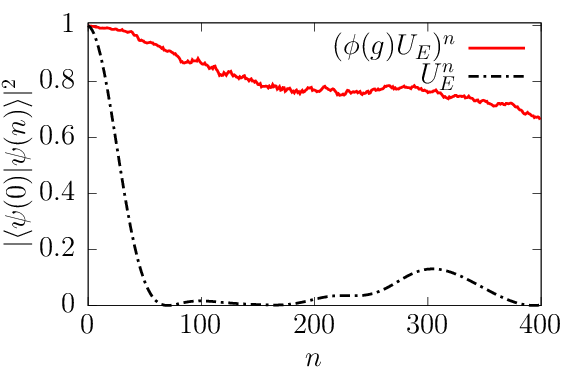# Near-term targets?

Inelastic scattering: need a $$(4\;\mathrm{fm})^3$$ box (at least). (See: Jordan, Lee, Preskill (2011).)

With a lattice spacing of $$0.1\;\mathrm{fm}$$, that's $$2 \times 10^5$$ links, or $$2 \times 10^6$$ qubits. Yikes!

What can we do with a small volume? Hydrodynamics

Fireballs

Early in a heavy-ion collision, temperature is $$\sim 300\;\mathrm{MeV}$$; physics well-described by hydrodynamics. $$\def\d{\mathrm{d}} \rho \frac{\d u_i}{\d t} + \partial_i p = {\color{blue}\eta} \left(\frac 1 3 \partial_i \partial_j u_j + \partial_j^2 u_i \right) + {\color{blue}\zeta}\cdots$$

The fireball is small ($$\sim 10\;\mathrm{fm}$$)! This is good for us: even smaller volumes might behave hydrodynamically.

EFT coefficients (shear viscosity, ...) not known from first principles.

# Preparing a thermal state

(The least sophisticated algorithm on the planet.)

Too cold? "Whack and wait".

Too hot? You're on your own.

Can we go cheaper?

# Avoiding state preparation

State preparation dominates the gate cost of the calculation.

Can we skip state preparation?

Classical lattice methods are very good at simulating thermodynamics; can't do real-time.

Quantum computers simulate real-time evolution easily; thermodynamics can be expensive.

Hybrid algorithm: thermodynamics classically, evolution quantumly

# The Euclidean lattice

Classical thermodynamics

Boltzmann factor $$p_i \propto e^{-\beta E_i}$$

Partition function $$Z = \sum_i e^{-\beta E_i}$$

Expectation values $$\langle \mathcal O\rangle = \sum_i p_i \mathcal O_i$$

Quantum thermodynamics

Density matrix $$\rho = e^{-\beta H}$$

Partition function $$Z = \mathrm{Tr\,}\rho$$

Expectation values $$\langle \mathcal O\rangle = Z^{-1} \mathrm{Tr\,} \mathcal O \rho$$

Several ways of turning $$\rho$$ into a probability distribution to sample. We could take the diagonal $$\langle \mathcal O \rangle = \frac{\sum_i \rho_{ii} \mathcal O_{ii}}{\sum_i \rho_{ii}}$$

Equality holds when $$\mathcal O$$ is diagonal. (Common in lattice QCD.)

# The real-time oracle

We want $$\langle \mathcal O(t)\rangle$$; the operator isn't diagonal! $$\langle \mathcal O(t)\rangle = \frac{\sum_{ij} \rho_{ij} \mathcal O(t)_{ji}}{\sum_{i}\rho_{ii}} = \left(\frac{\sum_{ij} \rho_{ij} \mathcal O(t)_{ji}}{\sum_{ij} \rho_{ij}}\right)\color{red}\underbrace{\left(\frac{\sum_{ij} \rho_{ij}}{\sum_{i} \rho_{ii}}\right)}_{\mathrm{Normalization}}$$

Therefore: sample pairs of states $$|i\rangle\langle j|$$, distributed by $$\rho_{ij}$$.

How to compute $$\mathcal O(t)_{ij}$$? This part is done on a quantum computer.

Preparing a basis state $$|i\rangle$$ is cheap. Start with the states $$|+\rangle = |i\rangle + |j\rangle\;\mathrm{ and }\; |-\rangle = |i\rangle - |j\rangle$$ and look at $$\langle + | \mathcal O(t) | +\rangle - \langle - | \mathcal O(t) | -\rangle$$.

# How to sample

How do we sample $$\rho_{ii}$$?

Even computing $$\rho_{ij}$$ is hard! Turns out, sampling is easier: $$\mathrm{Tr\,} e^{-\beta H} = \sum_{i,j,k,\ldots} \color{green}\left(e^{-\delta H}\right)_{ij} \left(e^{-\delta H}\right)_{jk} \cdots$$

For small $$\delta$$, the summand is easily computed. This is a joint distribution over $$(i,j,k,\ldots)$$. Marginalized, it approximates $$\rho_{ii}$$.

Diagonal operators $$\rho_{ii} \approx \left(e^{-\delta H}\right)_{ij} \left(e^{-\delta H}\right)_{jk} \left(e^{-\delta H}\right)_{ki}$$

(Periodic boundary conditions)

Arbitrary operators $$\rho_{i\ell} \approx \left(e^{-\delta H}\right)_{ij} \left(e^{-\delta H}\right)_{jk} \left(e^{-\delta H}\right)_{k\ell}$$

(Open boundary conditions)

# How much do we save?

One step of time evolution requires $$O(V)$$ operations.

With state prep, need to evolve for $$O(T + \Delta^{-2})$$ steps.

Characteristically, $$\Delta \sim V^{1/3}$$. Per measurement: $$\color{red} O(V^{5/3})$$

Without state prep, each quantum computation is now $$\color{green} O(V T)$$

At the cost of needing to perform many more computations (sampling $$\rho$$).

A big advantage for noisy quantum processors.

# The catch

Signal-to-noise problem!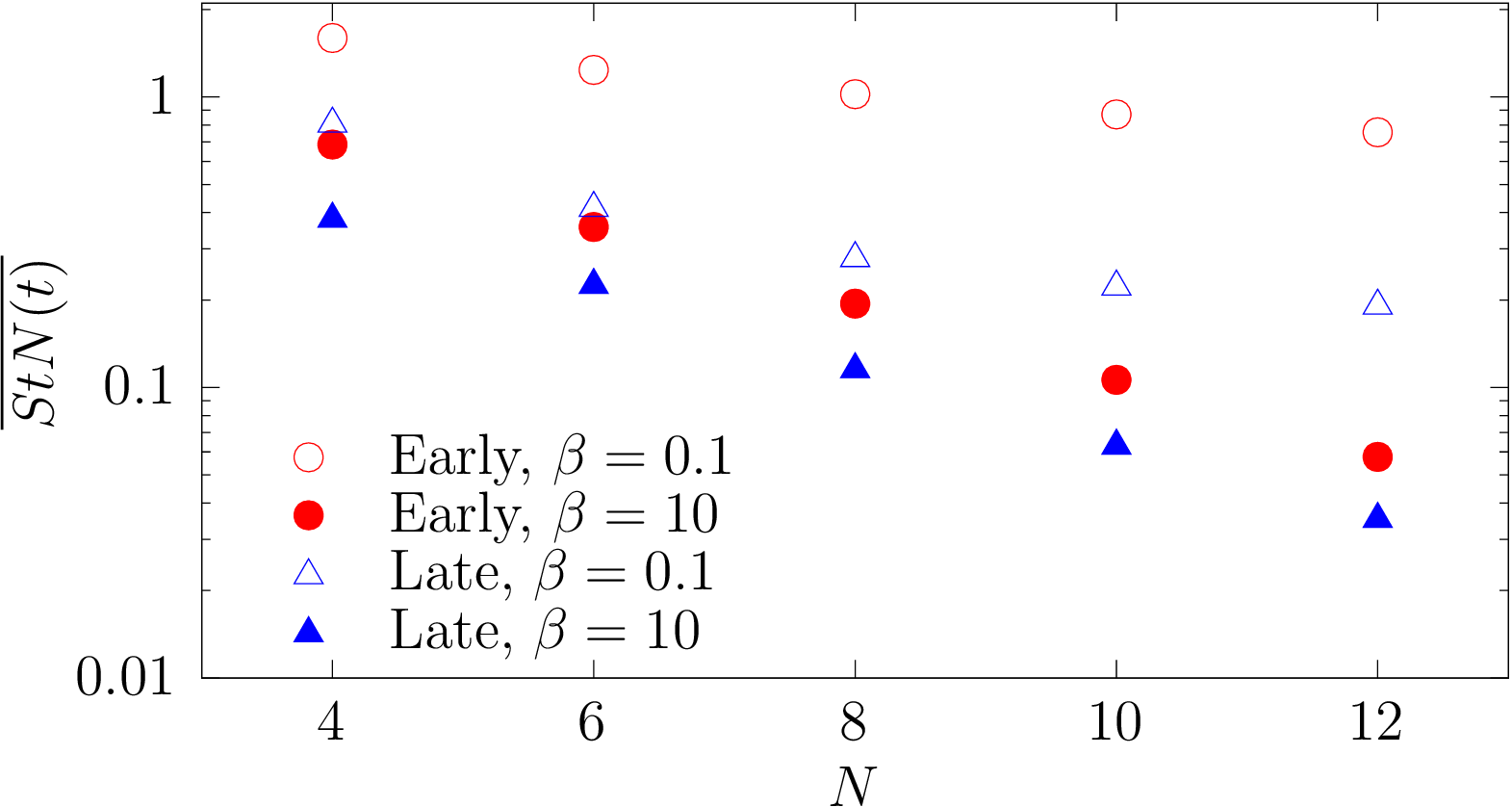$$\langle (\mathcal O_{ij})^2\rangle \gg \langle (\mathcal O)_{ij}\rangle^2$$

The QC calculation is shorter, but we need many of them.

Probably only useful for near-term, noisy processors.

# Measuring viscosity

Green-Kubo
$$\eta = \frac{\beta}{V} \int_0^{\infty} \mathrm{d} t \;\langle T^{12}(t) T^{12}(0)\rangle$$
Shear Wave
$$\def\d{\mathrm{d}} \rho \frac{\d u_i}{\d t} + \partial_i p = {\color{blue}\eta} \left(\frac 1 3 \partial_i \partial_j u_j + \partial_j^2 u_i \right) + {\color{blue}\zeta}\cdots$$
Create a shear wave: $u_x = A \cos(k z)$ Near equilibrium, a shear wave decays as $A(t) \sim A(0) e^{-\frac{\eta k^2}{\rho} t}$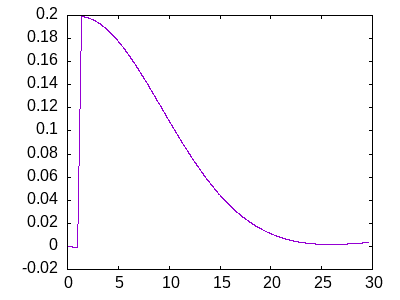(Discussed and endorsed by Hess (2001))

# Finite volume effects

Classical Approximation

Finite-volume effects set by $$\ell_{\mathrm{mfp}} \sim 0.5\;\mathrm{fm}$$. (Not trustworthy!)

Suggests box sizes with $$L \sim 1-2\;\mathrm{fm}$$ sufficient!

With a lattice spacing of $$0.2\;\mathrm{fm}$$, this is a $$5^3$$ to $$10^3$$ lattice.

Gauge/Gravity

Using Green-Kubo ($$T^{12}$$ channel, there are no finite-volume effects in the $$N_C \rightarrow \infty$$ limit of $$\mathcal N=4$$ SYM. (This is a holography special.)

In the $$T^{01}$$ channel, FV regulated by temperature. Again, the scale is $$\sim 1 \;\mathrm{fm}$$.

Stay tuned...

All told, for $$3 + 1$$ $$SU(3)$$ Yang-Mills, $$\sim 4 \times 10^4$$ qubits

For $$2 + 1$$ $$SU(2)$$ gauge theory, $$\lesssim 1500$$ qubits

Hydrodynamics of a (strongly interacting) $$2+1$$ spin model could be $$\sim 100$$ qubits.

Thanks for watching!
Questions?
/
< >